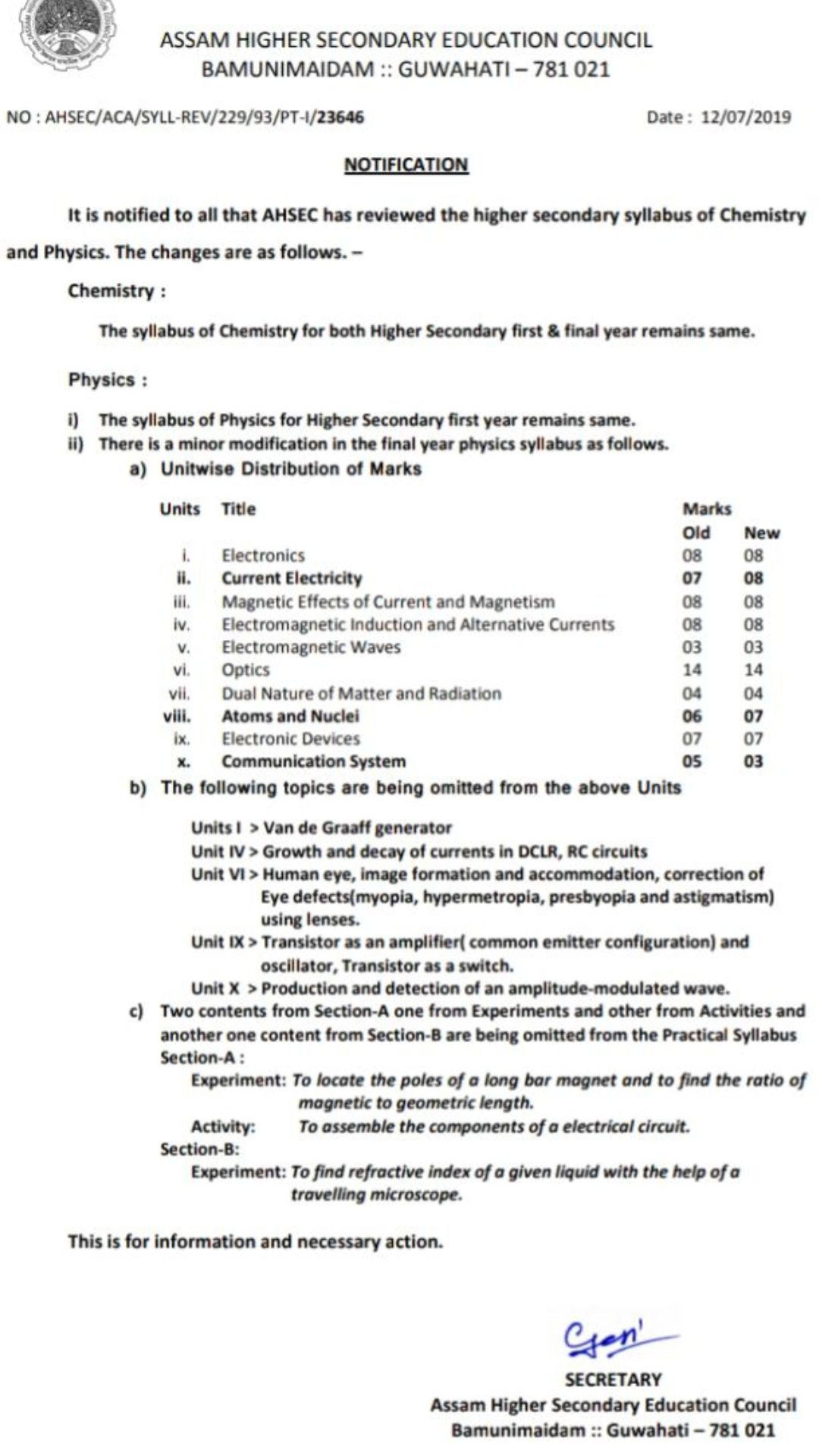## HS XII Electrostatics year-wise questions

2019

1. Mention one similarity between Coulomb force and gravitational force acting  between two stationary charges. [Mark-1]
2. Define one coulomb charge. Two point charges at a distance r in air exert a force F on each other. At what distance will these charges experience the same force F in a medium of dielectric constant k ? [Mark-1+1=2]
3. There is an electric dipole on the x-y plane. Its dipole moment is 4 x 10-9Cm . On the same plane there is also a uniform electric field  of magnitude 5 x 104 N/C . If the axis of the dipole makes an angle 300 with the electric field, calculate the magnitude ot the torque acting on the dipole and also mention the direction of torque [Mark-2+1=3]
4. The capacity of a parallel plate capacitor with air is 18 pF.  When a dielectric material is inserted in the space between the plates, its capacity becomes  108 pF. Calculate the permittivity of the material. What is the material ?  [Mark-2+1=3]

2018

1. Which experiment established the fact that electric charge is quantized ? [Mark-1]
2. Give the statement of Coulomb’s law for the force between two point charges. Write this law in vector notation for two point charges of same magnitude and opposite sign separated by a distance r in vacuum. [Mark-1+1=2]          (or)
3. Two point charges 0.01/μC and -0.01/μC are placed 10cm apart in vacuum. Calculate the magnitude of electric field intensity at the middle point of the line joining the charges and mention its direction.           [Mark-1+1=2]
4. Apply Gauss’s law to derive the expression for electric field intensity due to an infinitely long straight uniformly charged wire. What is the direction of the field intensity if it is positively charged ?                     [Mark-2+1=3]       (or)
5. Derive the expression for field intensity due to an electric dipole in vacuum for points on its axis. Compare the variation of field intensity with distance for an electric dipole and a point charge when both are kept in vacuum. [Mark-2+1=3]
6. A 600μF capacitor is charged by a 200V supply. It is then disconnected from the supply and is connected to another uncharged 600μF capacitor. How much electrostatic energy is lost in the process ?    [Mark-3]
7. In the diagram below , a particular of mass  “m” and charge (-q) initially moving along X-axis with velocity “vx” enters the region between two charged plates. The length of the plate system is “L” and uniform electric field between the plates is “E”. What is the vertical deflection of the particle at the far edge of the plate ? [Mark-3]

2017

1.      The product of permeability of free space and permitivity is —
(Choose the right option)
(i) c              (ii) c-1              (iiij c2                (iv )c-2    where c is velocity of light. [Mark-1]
2. In an electric field a unit positive charge is displaced from one point to another point along a straight line of length 2 cm and the work done is 2mJ . If it is displaced along a parabolic path . between the same points of length 5 cm, what will be the work done ?    [Mark-1]
3. Three electric point charges qo  ,  q1 and q2 are at distances r0, r and r2  respectively with respect to same origin. What is the force on charge q0 in the field of charges q1 and q2 ?[Mark-2]
4. The volume charge density within a volume Vis ρ(r). What is the force on a small test charge q0 placed outside the volume having position vector r0 with respect to the same origin considered to specify the position vector of the charge distribution within the volume. [Mark-2]
5. What is the net electric flux through a closed surface surrounding an electric dipole ? Derive the expressions for electric field intensity both inside and outside a uniformly charged spherical cell. What is the total charge enclosed by a closed surface if the electric flux entering and leaving the
surface are 20000 N/Cm2 and 30000 N/Cm2 respectively. Given∈0= 8.85x 10-12 C2N~1 m 2 .   [Mark-1+3+1=5]                             (or)
6. Why is an insulator sometimes called a dielectric ? What is the main difference between free and bound charge ? Generally one of the two plates of a Capacitor System is earthed, why? Derive the expression for the energy stored in a charged capacitor.           [Mark-1+1+1+2=5]

## Marking pattern for AHSEC Physics ,2019

Assam higher secondary education council announced new marking pattern for the subject physics 2019 . Marking pattern will be same for unit 1 ,unit 3 , unit 4, unit 5, unit 6, unit 7 and unit 9 . For unit 2 and unit 8 , 1 mark is increased  and for unit 10 2 mark is  decreased . Full marking scheme is given below

.ncert_xi_11